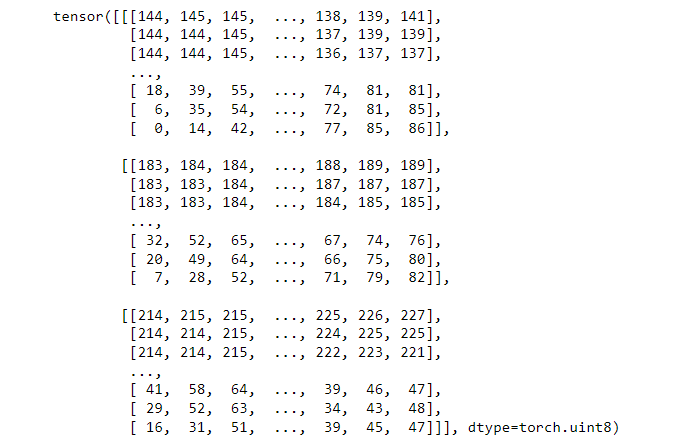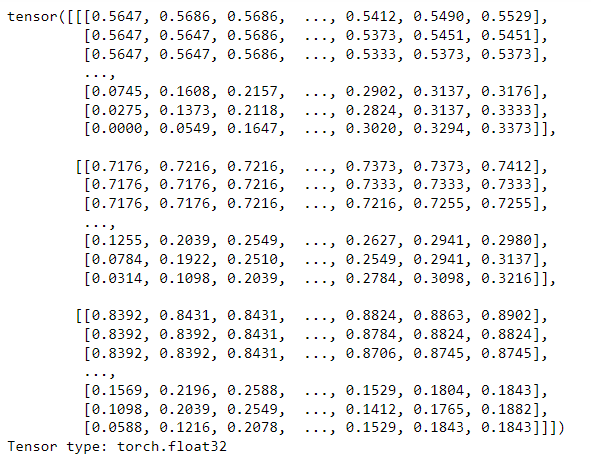# Converting an image to a Torch Tensor in Python

• Last Updated : 26 Jan, 2022

In this article, we will see how to convert an image to a PyTorch Tensor. A tensor in PyTorch is like a NumPy array containing elements of the same dtypes.

A tensor may be of scalar type, one-dimensional or multi-dimensional. To convert an image to a tensor in PyTorch we use PILToTensor() and ToTensor() transforms. These transforms are provided in the torchvision.transforms package. Using these transforms we can convert a PIL image or a numpy.ndarray. The numpy.ndarray must be in [H, W, C] format, where H, W, and C are the height, width, and a number of channels of the image.

transform = transforms.Compose([transforms.PILToTensor()])

tensor = transform(img)

This transform converts a PIL image to a tensor of data type torch.uint8 in the range between 0 and 255. Here img is a PIL image.

transform = transforms.Compose([transforms.ToTensor()])

tensor = transform(img)

This transform converts any numpy.ndarray to torch tensor of data type torch.float32 in range 0 and 1. Here img is a numpy.ndarray.

Approach:

• Import the required libraries.
• Read the input image. The input image is either PIL image or a NumPy N-dimensional array.
• Define the transform to convert the image to Torch Tensor.  We define a transform using transforms.Compose(). You can directly use transforms.PILToTensor() or  transforms.ToTensor().
• Convert the image to tensor using the above-defined transform.
• Print the tensor values.

The below image is used as an input image in both examples:### Example 1:

In the below example, we convert a PIL image to Torch Tensor.

## Python3

 `# Import necessary libraries``import` `torch``from` `PIL ``import` `Image``import` `torchvision.transforms as transforms`` ` `# Read a PIL image``image ``=` `Image.``open``(``'iceland.jpg'``)`` ` `# Define a transform to convert PIL ``# image to a Torch tensor``transform ``=` `transforms.Compose([``    ``transforms.PILToTensor()``])`` ` `# transform = transforms.PILToTensor()``# Convert the PIL image to Torch tensor``img_tensor ``=` `transform(image)`` ` `# print the converted Torch tensor``print``(img_tensor)`

Output:Notice that the data type of the output tensor is torch.uint8 and the values are in range [0,255].

### Example 2:

In this example, we read an RGB image using OpenCV. The type of image read using OpenCV is numpy.ndarray. We convert it to a torch tensor using the transform ToTensor()

## Python3

 `# Import required libraries``import` `torch``import` `cv2``import` `torchvision.transforms as transforms`` ` `# Read the image``image ``=` `cv2.imread(``'iceland.jpg'``)`` ` `# Convert BGR image to RGB image``image ``=` `cv2.cvtColor(image, cv2.COLOR_BGR2RGB)`` ` `# Define a transform to convert``# the image to torch tensor``transform ``=` `transforms.Compose([``    ``transforms.ToTensor()``])`` ` `# Convert the image to Torch tensor``tensor ``=` `transform(image)`` ` `# print the converted image tensor``print``(tensor)`

Output:Notice that the data type of the output tensor is torch.float32 and the values are in the range [0, 1].

My Personal Notes arrow_drop_up